# High School Chemistry : Thermochemistry and Energetics

## Example Questions

← Previous 1 3

### Example Question #1 : Help With Endothermic And Exothermic Reactions

What can be said about the rates of exothermic and endothermic reactions?

Possible Answers:

Exothermic reactions are more common than endothermic reactions

Endothermic reactions are more common than exothermic reactions

The rates of exothermic reactions are faster than the rates of endothermic reactions

None of these are valid answers

The rates of endothermic reactions are faster than the rates of exothermic reactions

Correct answer:

None of these are valid answers

Explanation:

The definition of an endothermic reaction is that the products have higher energy than the reactants, resulting in a positive enthalpy of reaction. For the reaction to run, there must be an input of energy. The opposite is true for exothermic reactions: the products have lower energy than the reactants, enthalpy of reaction is negative, and heat is released.

Nothing is can be definitively stated about the rates of either type of reaction without additional information, as that will depend on the specific reactions and their respective activation energies.

### Example Question #2 : Help With Endothermic And Exothermic Reactions

Which of the following is an example of an exothermic reaction?

Possible Answers:

Dry ice becoming gaseous carbon dioxide

Ice melting

Water boiling

Magma cooling to form igneous rocks

Correct answer:

Magma cooling to form igneous rocks

Explanation:

In an exothermic reaction, heat has been released to the surroundings from the system. As a result, the molecules are at a lower final energy state after releasing the energy to the surroundings.

Going from a solid to a gas (as well as liquid in between) is an endothermic reaction. Energy must be absorbed in order to raise the energy of the molecules so that the phase change can take place. Boiling water, melting ice, or sublimating carbon dioxide all require an input of energy.

The opposite is observed when magma cools. The liquid magma releases energy to the surroundings, allowing it to cool and form igneous rock. The magma is essentially "freezing," turning from a liquid to a solid.

### Example Question #3 : Help With Endothermic And Exothermic Reactions

Which of the following statements is true?

Possible Answers:

Condensation of steam is an endothermic reaction

Exothermic reactions have a lower activation energy compared to the reverse endothermic reactions

Only endothermic reactions require energy in order to take place

Endothermic reactions result in products with a lower internal energy

Correct answer:

Exothermic reactions have a lower activation energy compared to the reverse endothermic reactions

Explanation:

When graphically tracking the energy of a reaction, you can see that energy is always needed to start a reaction, regardless of its enthalpy. This necessary energy is called the activation energy. Exothermic reactions, however, have a lower activation energy compared to the reverse endothermic reaction. This is because there is a net energy release from an exothermic reaction because the products have less energy than the reactants. To reverse this reaction would be to go from the low energy products back to the high energy reactants, resulting in a net increase in energy (an endothermic process).

Condensation of steam is an exothermic process. Heat must be released since the high energy steam is becoming lower energy water.

### Example Question #4 : Help With Endothermic And Exothermic Reactions

The combustion of propane gas in a camping stove is an example of what type of reaction?

Possible Answers:

Endothermic reaction, with heat being released

Exothermic reaction, with heat being released

Endothermic reaction, with heat being absorbed

Exothermic reaction, with heat being absorbed

It depends on the temperature at which the reaction occurs

Correct answer:

Exothermic reaction, with heat being released

Explanation:

Combustion reactions occur when a compound is oxidized in a highly exothermic reaction. Most commonly, the reactant is a hydrocarbon (such as propane) and the oxidizing agent is oxygen gas. The result is a large release of heat energy, frequently visualized as a flame.

Note that exothermic reactions by definition release heat, while endothermic reactions absorb heat.

### Example Question #5 : Help With Endothermic And Exothermic Reactions

Which condition is always true for an exothermic reaction?

Possible Answers:

There must be a catalyst involved

The molecular bonds of the compound are being broken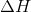is negative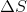is positive

Heat is being gained from the environment

Correct answer:is negative

Explanation:

When a reaction is exothermic ("exo-" meaning out and "-thermic" having to do with heat), it means that the reaction is giving off heat into the environment. Therefore, the reactants have a net heat loss throughout the process of the reaction.

The change in enthalpy,, is a measure of the change in heat energy during a reaction.is always negative for an exothermic process because the products always have less heat energy than the reactants.

### Example Question #6 : Help With Endothermic And Exothermic Reactions

Which condition is always true for an exothermic reaction?

Possible Answers:

Heat is being gained from the environmentis positive

There must be a catalyst involvedis negative

Correct answer:is negative

Explanation:

When a reaction is exothermic ("exo-" meaning out and "-thermic" having to do with heat), it means that the reaction is giving off heat into the environment. Therefore, the reactants have a net heat loss throughout the process of the reaction.

The change in enthalpy,, is a measure of the change in heat energy during a reaction.is always negative for an exothermic process because the products always have less heat energy than the reactants.

### Example Question #7 : Help With Endothermic And Exothermic Reactions

Which condition is always true for an exothermic reaction?

Possible Answers:is negative

Heat is being gained from the environment

There must be a catalyst involvedis positive

Correct answer:is negative

Explanation:

When a reaction is exothermic ("exo-" meaning out and "-thermic" having to do with heat), it means that the reaction is giving off heat into the environment. Therefore, the reactants have a net heat loss throughout the process of the reaction.

The change in enthalpy,, is a measure of the change in heat energy during a reaction.is always negative for an exothermic process because the products always have less heat energy than the reactants.

### Example Question #1 : Thermochemistry And Energetics

Which of the following is considered an exothermic reaction?

Possible Answers:

Forming a bond

Single-replacement reactions

Decomposition reactions

Breaking a bond

Synthesis reactions

Correct answer:

Forming a bond

Explanation:

An exothermic reaction releases energy into the environment. In contrast, endothermic reactions require an input of energy to initiate the reaction.

Forming a bond is always an exothermic reaction because it releases energy. Breaking a bond always requires energy, and is thus an endothermic process. Synthesis, decomposition, and single-replacement reactions can be either exothermic or endothermic, and cannot be determined without more information.

### Example Question #2 : Thermochemistry And Energetics

Which type of reaction will happen spontaneously?

Possible Answers:

Exothermic reactions

Reactions that increase entropy

Endothermic reactions

Exergonic reactions

Endergonic reactions

Correct answer:

Exergonic reactions

Explanation:

Spontaneity is determined by Gibbs free energy. When Gibbs free energy is less than zero, the reaction is considered exergonic and will occur spontaneously. When Gibbs free energy is greater than zero, the reaction is considered endergonic and will not occur spontaneously.

Exothermic reactions cause a release of enthalpy (heat) from the system and endothermic reactions require and input of energy to initiate the reaction. Gibbs free energy is determined by enthalpy, entropy, and temperature. A negative enthalpy, high temperature, and high entropy will cause the reaction to be more spontaneous, but must all come together to contribute. Simply because a reaction is exothermic does not meant that is increases entropy enough to be spontaneous.

### Example Question #1 : Help With Enthalpy

Consider the following balanced reaction: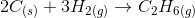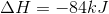What is the change in enthalpy if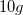of solid carbon is used in the above reaction?

Possible Answers: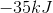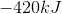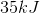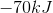Correct answer:Explanation:

The enthalpy of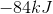describes the amount of heat when the amount of carbon in the balanced reaction (two moles) is used. Since onlyof carbon are used, we can find how much heat is released.

When two moles of carbon are used,are released. Two moles of carbon is equal to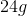of carbon, based on carbon's atomic mass.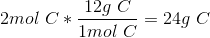Knowing this, we can set up proportions in order to determine how much heat is released byof carbon.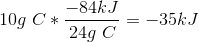Soof carbon results inof heat being released to the surroundings.

← Previous 1 3

### All High School Chemistry Resources